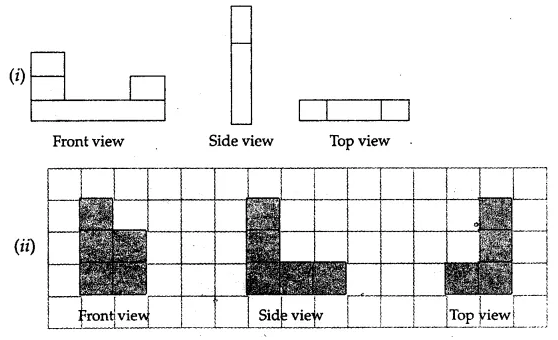# ML Aggarwal Class 7 Solutions for ICSE Maths Chapter 15 Visualising Solid Shapes Ex 15.3

## ML Aggarwal Class 7 Solutions for ICSE Maths Chapter 15 Visualising Solid Shapes Ex 15.3

Question 1.
Count the number of cubes in the following structures: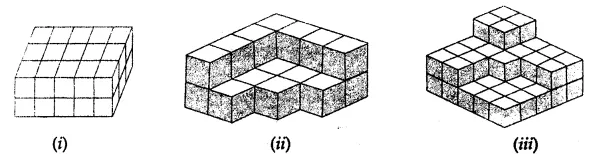Solution: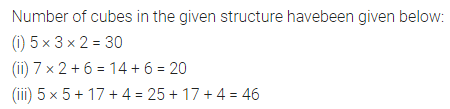Question 2.
What cross-section is made in the following
(i) vertical cut (ii) horizontal cut?
(a) A brick
(b) A round apple
(c) A die
(d) A circular pipe
(e) An ice cream cone
(f) A square pyramid
Solution: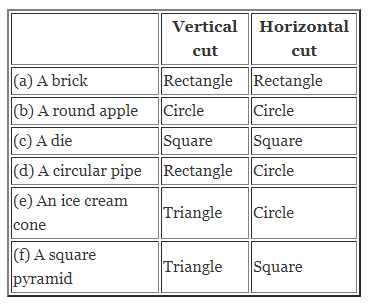Question 3.
For each solid given below, the three views (1), (2) and (3) are given. Identify for each solid the corresponding top, front and side views: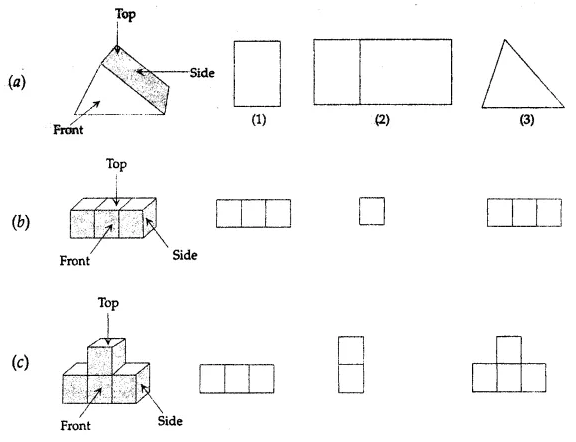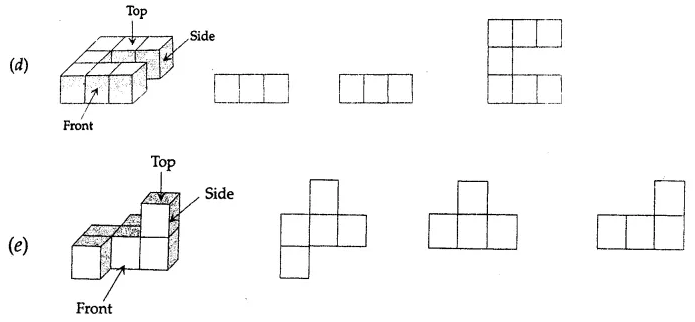Solution: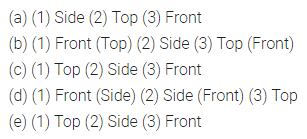Question 4.
For the solids given below sketch the front, side and top view:Solution: